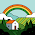## Wednesday, May 17, 2017

### codefights smooth sailing ( CommonCharacterCount)

`https://codefights.com/arcade/intro/level-3/JKKuHJknZNj4YGL32`
```
```
```public static int commonCharacterCount(String s1, String s2) {
int sum = 0;

char[] as= s1.toCharArray();
char[] bs= s2.toCharArray();

int[] ias = new int;
int[] ibs = new int;

for (int i = 0; i < as.length; i++) {
ias[(int)as[i]]++;
}

for (int i = 0; i < bs.length; i++) {
ibs[(int)bs[i]]++;
}

for (int i = 0; i < ibs.length; i++) {
sum += Math.min(ias[i], ibs[i]);
}
return sum;
}```

Given two strings, find the number of common characters between them.
Example
For `s1 = "aabcc"` and `s2 = "adcaa"`, the output should be
`commonCharacterCount(s1, s2) = 3`.
Strings have `3` common characters - `2` "a"s and `1` "c".
Input/Output
• [time limit] 3000ms (java)
• [input] string s1
A string consisting of lowercase latin letters `a-z`.
Guaranteed constraints:
`1 ≤ s1.length ≤ 15`.
• [input] string s2
A string consisting of lowercase latin letters `a-z`.
Guaranteed constraints:
`1 ≤ s2.length ≤ 15`.
• [output] integer

#### 2 comments:

1.Question for you, and hopefully you respond. Why did you set the ias ibs arrays to int? Was it to hold all the ascii numbers?

1.just thought about 126 include a-z ;;

but only actually 26 size of array needed

maybe next time i will make int